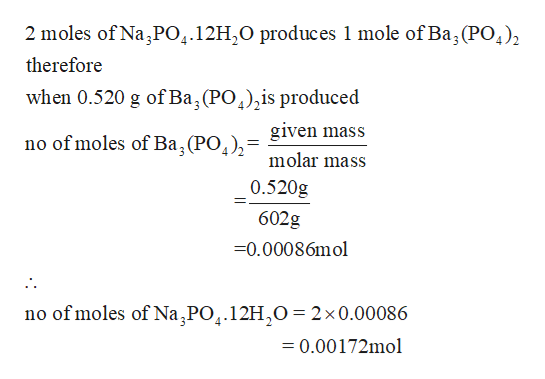# a 1.500 g sample of a mixture of solid Na3PO4 *12H2O and BaCL2* 2H2O was dissolved in water and formed 0.520g of Ba3PO4 precipitate . If reagent tests demonstrated that Na3PO4* 12H2O was the limitng reactant in the precipitaion reaction, calculate the mass percent of each reactant salt in the original sample.

Question
40 views

a 1.500 g sample of a mixture of solid Na3PO4 *12H2O and BaCL2* 2H2O was dissolved in water and formed 0.520g of Ba3PO4 precipitate . If reagent tests demonstrated that Na3PO4* 12H2O was the limitng reactant in the precipitaion reaction, calculate the mass percent of each reactant salt in the original sample.

check_circle

Step 1

The reaction proceeds according to the following equation

Step 2

Since Na3PO4.H2O is the limiting reagenthelp_outlineImage Transcriptionclose2 moles of Na,PO4.12H,0 produces 1 mole of Ba,(PO4)2 therefore when 0.520 g of Ba3 (PO4),is produced given mass no of moles of Ba^ (PO4)2- molar mass 0.520g 602g 0.00086mol no of moles of Na^PO4.12H,0= 2x 0.00086 =0.00172mol fullscreen
Step 3

Mass of Na3PO4.H2O

...

### Want to see the full answer?

See Solution

#### Want to see this answer and more?

Solutions are written by subject experts who are available 24/7. Questions are typically answered within 1 hour.*

See Solution
*Response times may vary by subject and question.
Tagged in

### Quantitative analysis